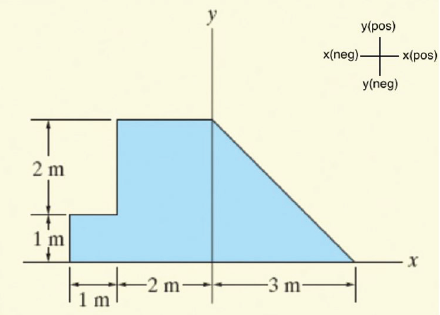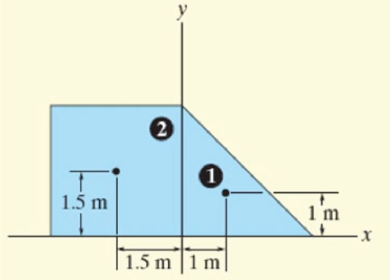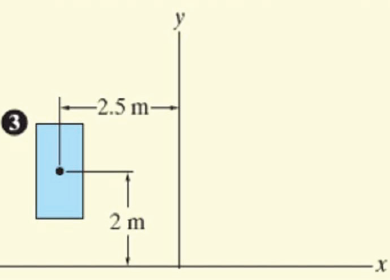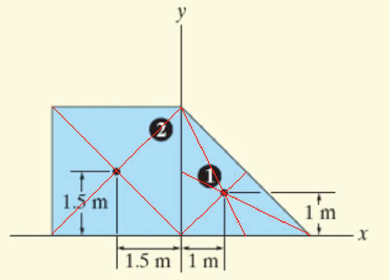### How to Determine the Center of Gravity of Any LoadFeared by many, understood by few, the center of gravity is the single most underestimated and undervalued property of a load. Since the vast majority of all transports and lifts comprise loads with a fairly centralized CoG there is not much incentive to dive into the theories of something that cannot be seen or measured with a tape.

If the CoG is not centralized, however, or the load is close to the limit of the transport or lift equipment, it becomes an increasingly more important property that can make or break the success of a project.

What is the center of gravity? In mathematical terms, “The center of gravity is the point at which an object’s entire mass can be assumed”. This may sound a bit abstract but with the below examples this will become clear. The CoG is not determined by shape or size, just by the weight and the location (coordinates) of each of its individual components.

It may be clear that the center of gravity of a square box is right in the center of that box and that “handling” that box, lifting, jacking, transporting etc., is not a complicated exercise from a mathematical point of view. That changes when it comes to dealing with objects that are built up from many individual items. Sooner or later everybody will get into this situation, where it becomes much more challenging. Such objects can be skids that contain components from different suppliers or, for example, vessels with varying diameters, oilers with a pressure vessel located on once site or just a wooden crate with unknown contents or whether the hand painted CoG mark can be trusted.

Finding out

Let’s start with a simple way of determining the CoG. This method can be applied to every object of which the CoG needs to be determined. Observe Figure 1, this is a two-dimensional object, for example, a steel plate with a random shape for which the CoG needs to be determined. This cannot be done in one step, but has to be performed is a series of steps.

Note: the distances are given in meters but this can be any unit of measure: feet, yards, etc. For the results of the calculation this makes no difference.

Step 1

Choose the location of the x-axis and the y-axis. These can be chosen in any location, the outcome of the calculation will remain the same if these axes are chosen in a different location. Try it.

For this example the x-axis is chosen at the base of the object and the y-axis is chosen so it runs from straight down between the triangular and rectangular shape.Step 2

Choose which side of the x-axis is the positive side and which one is the negative side. This is needed because a 1 meter distance from the y-axis could be on either side and without the “+” or “-“ designation there is no way of knowing this and, by definition, the outcome of the calculation will be wrong.

Step 3

We now need to divide the object into smaller “sub-objects” for which we can easily determine the CoG. This is shown in figure 2 and in figure 3. There is no real science behind choosing these smaller objects. Anything will do, as long as the total object is covered, obviously nothing should be forgotten or left out. In this example we have chosen:

1. A triangle (a 3 x 3 m square cut in half)
2. A square (3 x 3 m) note that this box includes a “void”
3. A rectangle (1 x 2 m) this is the void of the square mentioned above at 2Step 4

Of each of these sub-objects the CoG should be determined and expressed in co-ordinates. Observe figures 4 and 5. By drawing assist lines (shown in red) from one corner to the opposite corner the location of the CoG can be found for squares and rectangles. By drawing (assist) lines from one corner to the center of the opposite side the location of the CoG can be found for triangles. This CoG location is found at the intersection of these assist lines. The result is as follows:

CoG 1. (+1.0, +1.0)

CoG 2. (-1.5, +1.5)

Cog 3.  (-2.5, +2.0)